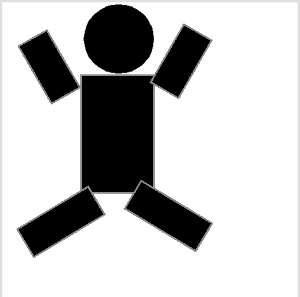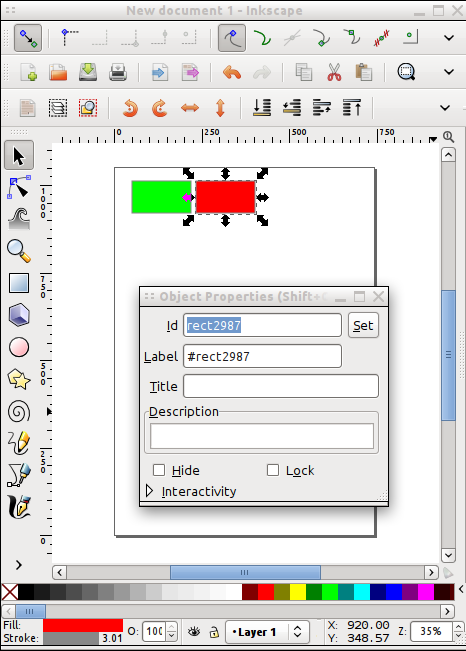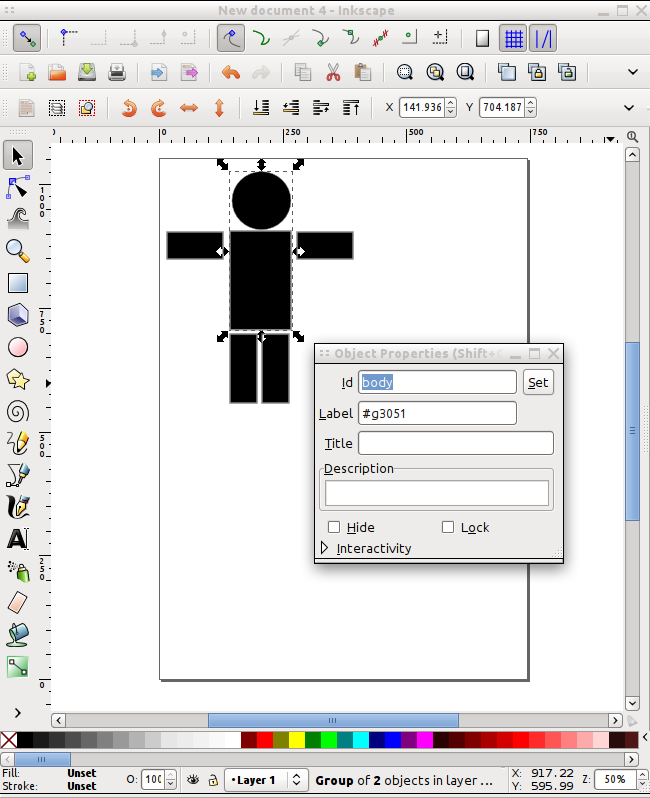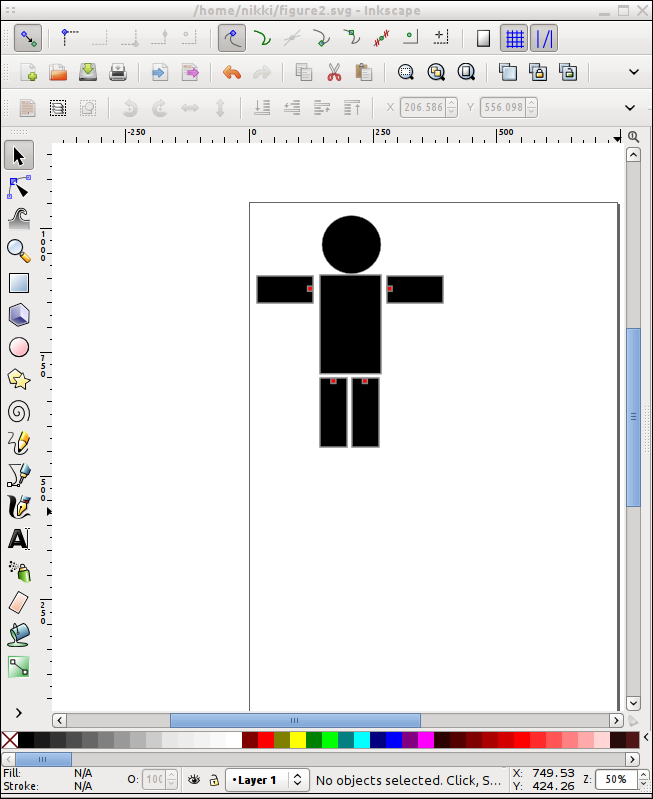## howto use svg for rigged 2D-animations in processing

I played around a bit with svg-files and processing and learned that the PShape-Class is far more powerfull that it looks on the first glace.

This is a short tutorial that shows how I used Inkscape and Processing to make a simple rigged 2D-figure.In processing loading and displaying svg-files is very easy. Just define a 'PShape' object and use 'loadShape' to fetch a svg-file. With the 'shape' command the graphic can be displayed in the window.

``````PShape boxes;

void setup() {
size(500,200);
}

void draw() {
background(255);
shape( boxes, 0, 0 );
}
``````

But when I looked at the source of the PShape class I learned that you can do far cooler stuff with these svg graphics. Every part of the svg graphics can be accessed by using the 'getChild'-method of the PShape class. So we could give the rectangles from the previous example a name and then hide them individually.

In inkscape for example right-click on an element, select the properties dialog and edit the 'id'-element. In this example named the boxes 'red_box' and 'green_box'To hide the red box for example we can use 'getChild(\"red_box\")' to select the red box and then use "setVisible(false)" to hide it.

``````PShape boxes;

void setup() {
size(500,200);
boxes.getChild( "red_box").setVisible(false);
}

void draw() {
background(255);
shape( boxes, 0, 0 );
}
``````

The Selected Child Nodes can not only be hidden but also scaled, rotated or translated separatly. So we are going to make a simple stick-figure in inkscape that will be turned into a jumping-jack.

I grouped the head and the body rectangle together and gave the group the id body because I didn't want to move the head separatly. I also had to convert the ellipse into a path, because processing didn't like the way inkscape saved the ellipse.To test if all the shapes are named correctly I tried to address and draw each element of the figure.

``````PShape fig;
PShape body;
PShape left_arm;
PShape right_arm;
PShape left_leg;
PShape right_leg;

void setup() {
size(500,500);
body = fig.getChild( "body" );
left_arm = fig.getChild( "left_arm" );
right_arm = fig.getChild( "right_arm" );
left_leg = fig.getChild( "left_leg" );
right_leg = fig.getChild( "right_leg" );

}

void draw() {
background(255);
shape( body, 0, 0 );
shape( left_arm, 0, 0 );
shape( right_arm, 0, 0 );
shape( left_leg, 0, 0 );
shape( right_leg, 0, 0 );

}
``````

Now I could use the 'rotate' command of each shape to rotate it, but the pivot-point for the rotation would be (0,0) - not quite what I want. So to find the right pivot-point I use small marker rectangles and group them together with the limp I want to rotate. I named the marker items 'pivot_left_arm', 'pivot_right_arm' and so on.To make them invisible I added the following lines to the setup method.

``````...
left_arm.getChild( "pivot_left_arm").setVisible( false );
right_arm.getChild( "pivot_right_arm").setVisible( false );
left_leg.getChild( "pivot_left_leg").setVisible( false );
right_leg.getChild( "pivot_right_leg").setVisible( false );
...
``````

To use the pivot point for rotating an arm for example, I can use the getParams() method to access the x and y coordinates of the small rectangle. Then I use translate to move the limb to the origin rotate it and move it back.

``````  ...
float[] p = left_arm.getChild( "pivot_left_arm" ).getParams();
float px = p;
float py = p;
left_arm.translate( px, py );
left_arm.rotate( a );
left_arm.translate( -px, -py );
....
``````

This rotations are additive and change the complete figure PShape object, so don't use it in the draw method, because otherwise the limp will start to rotate like a propeller.

In my final jumping-jack-Sketch I use to variables to remember the angle of the arms and legs and use a 'mouseMoved' method to rotate the limps.

``````PShape ofig;
PShape fig;

PShape body;
PShape left_arm;
PShape right_arm;
PShape left_leg;
PShape right_leg;

void setup() {
size(500,500);
body = fig.getChild( "body" );
left_arm = fig.getChild( "left_arm" );
right_arm = fig.getChild( "right_arm" );
left_leg = fig.getChild( "left_leg" );
right_leg = fig.getChild( "right_leg" );

left_arm.getChild( "pivot_left_arm").setVisible( false );
right_arm.getChild( "pivot_right_arm").setVisible( false );
left_leg.getChild( "pivot_left_leg").setVisible( false );
right_leg.getChild( "pivot_right_leg").setVisible( false );

}

void draw() {
background(255);

shape( fig, 0, 0 );

}

float r_arm = 0;
float r_leg = 0;

void mouseMoved() {
float a = radians(mouseY - pmouseY);

if ( r_arm+a < HALF_PI && r_arm + a  > -HALF_PI ) {
r_arm += a;

rotateShape( left_arm, "pivot_left_arm", a);
rotateShape( right_arm, "pivot_right_arm", -a);
}

if ( r_leg+a < 0 && r_leg + a  > -PI/3) {
r_leg += a;
rotateShape( left_leg, "pivot_left_leg", a);
rotateShape( right_leg, "pivot_right_leg", -a);

}
}

void rotateShape( PShape s, String pivot, float a ) {
float[] p = s.getChild( pivot ).getParams();
float px = p;
float py = p;
s.translate( px, py );
s.rotate( a );
s.translate( -px, -py );
}
``````